Search IntMath
Close

450+ Math Lessons written by Math Professors and Teachers

5 Million+ Students Helped Each Year

1200+ Articles Written by Math Educators and Enthusiasts

Simplifying and Teaching Math for Over 23 Years

# I heart math

By Murray Bourne, 04 Oct 2010

I saw this T-shirt recently.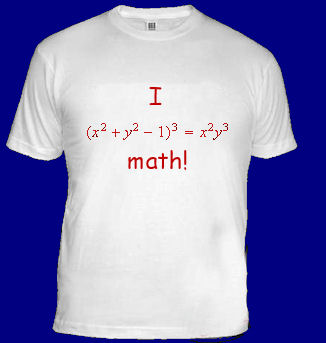What does it mean and what's that equation?

This is an example of an implicit function. When we first learn about functions, they are written explicitly, for example:

f(x) = sin(x) + 4x

This explicit function involves one dependent variable only and for each x value, we only get one f(x) value. Of course, I could also write this as

y = sin(x) + 4x

Notice y is on the left by itself, and the terms involving x are on the right, by themselves.

But there are many functions that are really messy when written explicitly, and so we turn to implicit functions.

In implicit functions, we see x's and y's multiplied and mixed together.

## A simple example

A simple example of an implicit function is the familiar equation of a circle:

x2 + y2 = 16

In this simple case, we can turn this into an explicit function by solving for y and getting 2 solutions: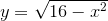or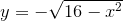But often it is very difficult, if not impossible, to solve an implicit function for y.

## The t-shirt Function

Returning to the t-shirt example, we have the implicit function:

(x2 + y2 − 1)3 = x2y3

We can expect more than one y-value for each x-value.

To graph it, we proceed as follows. Let's choose some easy values of x and y.

If x = 0, we substitute and obtain:

((0)2 + y2 − 1)3 = (0)2y3

(y2 − 1)3 = 0

We get 2 solutions, y = ± 1.

Now, let y = 0, and we get:

(x2 + (0)2 − 1)3 = x2(0)3

(x2 − 1)3 = 0

This gives us 2 solutions, x = ± 1.

So we know the curve passes through (-1, 0), (0, -1), (1, 0) and (0, -1),

Now, we choose some values of x between 0 and 1. We start with x = 0.2:

((0.2)2 + y2 − 1)3 = (0.2)2y3

This gives:

(-0.96 + y2)3 = 0.04y3

Solving this for y gives the real solutions: y = -0.824 or y = 1.166 (and 4 complex solutions).

We choose some more values and construct a table containing the real solutions:

 x y1 y2 0 0.2 0.4 0.6 0.8 1 1.2 -1 -0.824 -0.684 -0.520 -0.307 0 complex 1 1.166 1. 227 1. 231 1.17 complex

This equation is symmetrical, so we get the same correspnding values for -0.2, -0.4, -0.6, -0.8, -1 and -1.2.

In fact, outside of this range of x-values, there are no real y-values.

If we take a lot of points and join them, we get the following graph: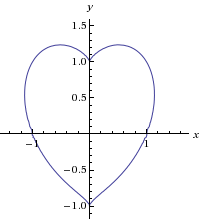So the t-shirt means "I heart math" (that is, "I love math").

## 3-D Example

Here's another one in 3 dimensions. The implicit function is: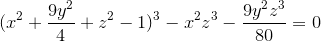for -3 ≤ x, y, z ≤ 3 (which means each of x, y and z takes values only between -3 and 3).

And here's the shirt: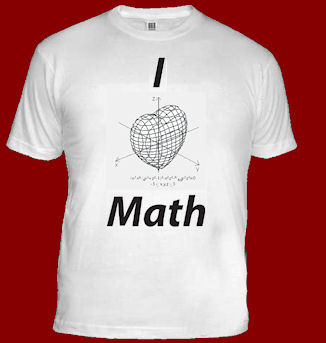Differentiation of implicit functions

Curves in polar coordinates

### 11 Comments on “I heart math”

1. Naren says:

This is really cool!. Your blog really helps me a lot. I love the t-shirt as well. Could I know where you found it?

2. vonjd says:

WA can do it too 🙂
http://www.wolframalpha.com

and then type:
plot (x^2 + y^2 - 1)^3 = x^2 y^3

3. Murray says:

Yes! The graph in the post comes from Wolfram|Alpha.

4. Samar says:

So marvelous explanation about the t shirt especially I am a math teacher
Thanks.

5. yasin.. says:

superb...nicely explained..!!!!!!!!!!!

6. musa says:

I like it!! To draw this graph i often use MATLAB.

7. Kudzai Maravanyika says:

i realy liked the way you express you maths. Keep on doing that.

8. Ryan says:

If you have a ti-89 you can graph the implicit 2d function in the 3d mode by typing in the equation and formatting the plot to make it graph implicitly. The window will have to be seriously adjusted though.

9. barbina says:

oh thank you I have been asked directly to Bagatrix and not precalculus graph sholved but must be solved to complete the curves and graphs, again thank you ...

11. april says:

wow!! amazing.. I like it..
thanks! i think i should suggest it to our math club as a batch shirt..
i like the graphing of the equation in 3-D.

### Comment Preview

HTML: You can use simple tags like <b>, <a href="...">, etc.

To enter math, you can can either:

1. Use simple calculator-like input in the following format (surround your math in backticks, or qq on tablet or phone):
a^2 = sqrt(b^2 + c^2)
(See more on ASCIIMath syntax); or
2. Use simple LaTeX in the following format. Surround your math with $$ and $$.
$$\int g dx = \sqrt{\frac{a}{b}}$$
(This is standard simple LaTeX.)

NOTE: You can mix both types of math entry in your comment.